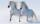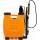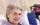Tablecloth

I embroider tablecloth in 20 days but if I embroider 3/4 hours a day I have embroider it for 15 days. How long do I embroider a day?

Result

x =  1 h

Solution:

3/4*20 = 15x

60x = 60

x = 1

Calculated by our simple equation calculator.

Leave us a comment of this math problem and its solution (i.e. if it is still somewhat unclear...):Be the first to comment!To solve this verbal math problem are needed these knowledge from mathematics:

Do you have a linear equation or system of equations and looking for its solution? Or do you have quadratic equation?

Next similar math problems:

1. Feed for horsesIn the kennel have feed for two horses for 21 days. The station brought an additional 5 horses. How many days will last the feed for all the horses if they have the same daily ration?
2. Construction of roadFor the construction of the road dam, it is supposed to transport six trucks a total of 18 days. After three days, two more cars were sent to the construction site. How long will it take to carry the material?
3. NovakNovak needed to dig up three of the same pit in the garden. The first pit dug father alone for 15 hours. His second dig son helped him and it did that in six hours. The third pit dug son himself. How long it took him?
4. SprayersThe vineyard can be sprayed first sprayer in 5 hours and with the second in 8 hours. How long would it take if we use sprayers both at once?
5. Pumps A and BPump A fill the tank for 12 minutes, pump B for 24 minutes. How long will take to fill the tank if he is only three minutes works A and then both pumps A and B?
6. Hectoliters of waterThe pool has a total of 126 hectoliters of water. The first pump draws 2.1 liters of water per second. A second pump pumps 3.5 liters of water per second. How long will it take both pumps to drain four-fifths of the water at the same time?
7. 1.5 divided1.5 divided by 1 = w divided by 4
8. FactoryIn the factory workers work in three shifts. In the first inning operates half of all employees in the second inning and a third in the third inning 200 employees. How many employees work at the factory?
9. Negative in equation2x + 3 + 7x = – 24, what is the value of x?
10. Simple equationSolve the following simple equation: 2. (4x + 3) = 2-5. (1-x)
11. Bed timeTiffany was 5 years old; her week night bedtime grew by ¼ hour each year. If, at age 18, her curfew time is 11pm, what was her bed time when she was 5 years old?
12. NormThree workers planted 3555 seedlings of tomatoes in one dey. First worked at the standard norm, the second planted 120 seedlings more and the third 135 seedlings more than the first worker. How many seedlings were standard norm?
13. Simple equationSolve for x: 3(x + 2) = x - 18
14. CakesGrandmother baked cakes. Half of its was poppy, quarter with plum jam and 16 cheesecakes. How many cakes she baked in total?
15. Forest nurseryIn the forest nursery after winter, they found that 1/10 stems died out of them. For them, they land 193 new spruces. How many spruces are in the forest nursery?
16. FamilyMom is 39 years old. Karl is eight years and Paul 4 years. For many years mother would old just like two children together?
17. Dropped sheetsThree consecutive sheets dropped from the book. The sum of the numbers on the pages of the dropped sheets is 273. What number has the last page of the dropped sheets?